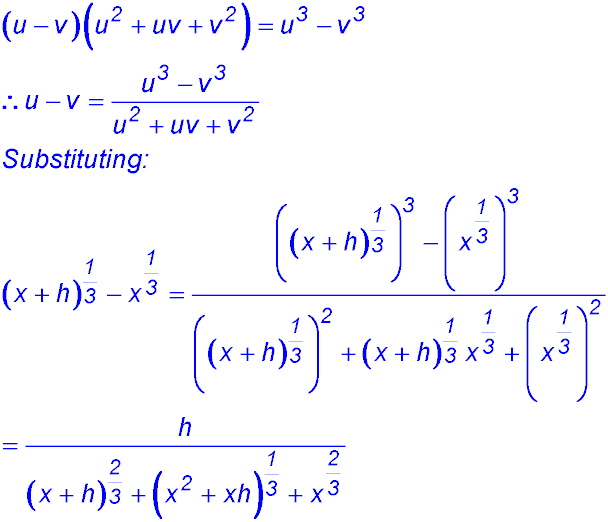Dr. J's Maths.
Where the techniques of Maths
are explained in simple terms.

Algebra - Indices and Powers.
Test yourself 3 - Solutions to the Advanced questions.

The following questions aim to help you evaluate your understanding of the five basic concepts underlying this topic. The questions are generally more difficult and require three or more steps to complete.
Use the HINTS given if you need to.

TECHNIQUE: Be systematic in your solving of these (indeed all) mathematics problems. In questions such as those below, be systemativ and move left to right by viewing the same types of terms. For example begin with collecting the numbers and then combine the first pronumeral terms together, then the second, etc.

Take your time and write out results at each step. Its better to take a few more seconds to monitor what you are doing than to rush and do too many steps at once and therefore make mistakes.

Simplify:

 1.2.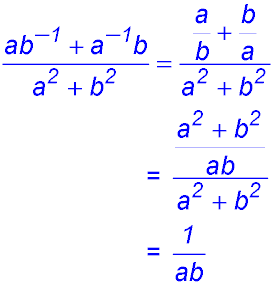3.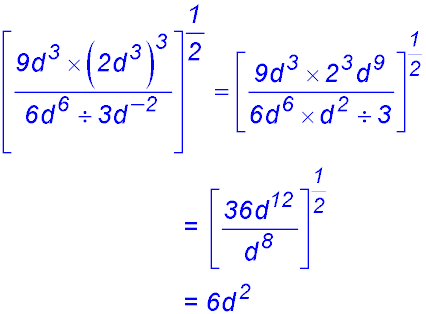4.5.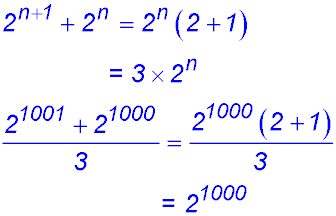6.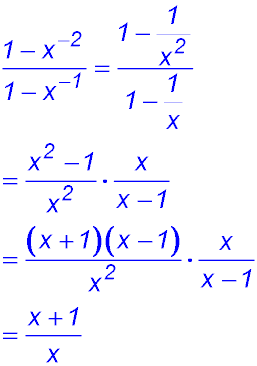Expand and simplify:

 7.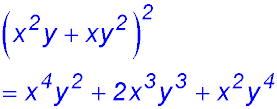8.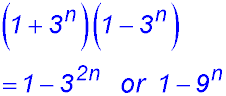9. Expand and simplify: (m + m-1)2 10. Expand and simplify: (2g-2f3)-2 × (2gf-3)4 11. Expand and simplify to prove: (3x)-3 × (32x)4 = 243x 12. If a = -3 and b = 5, compare the values for the expressions a3 - 2b2 and b3 - 2a2.

Substitution:
Do NOT just use your calculator to obtain the answer for the following questions. If you do, you will not be practicing the essential skills.

You will get the correct answer - but so what? What is really important? No one is watching - and you know.

The other problem is that I will haunt you with my voice coming back saying "you used it - didn't you"13. If x = 9 and y = 25: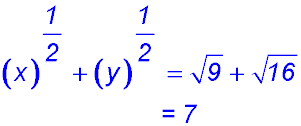14. If x = 32 and y = 24. Comment.Take this solution one step at a time. Retain the indices of 6 and 8 and convert the 27 and 4 into powers within the brackets. Then convert the powers into the basic given powers for x and y. Don't rush. 15.Comment.Easier to take the two powers for A and B and simplify them a little first. Then substitute into the required expression and simplify. 16.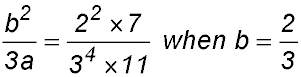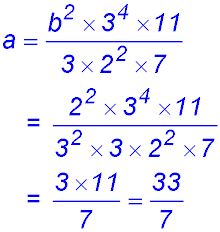Comment.There are a few ways to approach this question but it is probably easiest to begin by simply changing the subject of the equation to a. Then substitute for b and combine terms with like bases.

Solving equations:

The fundamental concept here is that if the bases of an equation are equal, the exponents must also be equal.

 17.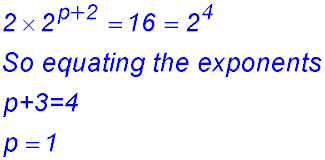18.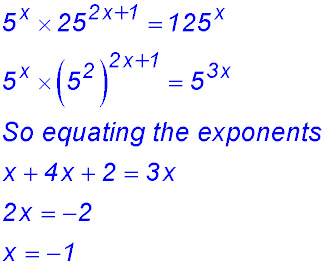19.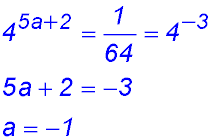20.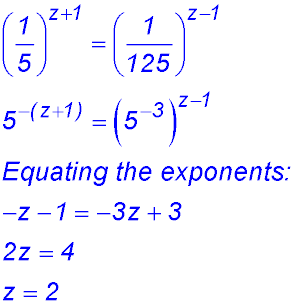21. Solve for x: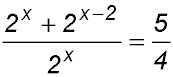22. Solve for a and b: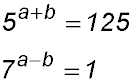29. (i)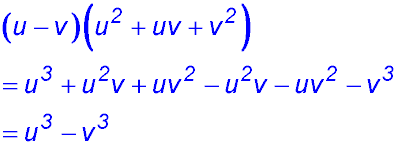(ii)